Courses

# Test: Measurement of Pressure

## 15 Questions MCQ Test Sensor & Industrial Instrumentation | Test: Measurement of Pressure

Description
This mock test of Test: Measurement of Pressure for GATE helps you for every GATE entrance exam. This contains 15 Multiple Choice Questions for GATE Test: Measurement of Pressure (mcq) to study with solutions a complete question bank. The solved questions answers in this Test: Measurement of Pressure quiz give you a good mix of easy questions and tough questions. GATE students definitely take this Test: Measurement of Pressure exercise for a better result in the exam. You can find other Test: Measurement of Pressure extra questions, long questions & short questions for GATE on EduRev as well by searching above.
QUESTION: 1

### The liquid in a manometer has a specific weight of 8.5 kN/m3. If the liquid rises 83 cm higher in the lower pressure leg, what is the difference in the pressure between the higher and the lower legs?​

Solution:

Δp = γ Δh = 8.5 × 83/100 kPa = 7.05 kPa

where γ is the specific weight of the liquid in the manometer and γ h is the difference of the heights.

QUESTION: 2

### What is the liquid density in a manometer, if the difference in the liquid levels in the manometer tubes is 1.35m, and the differential pressure between the tubes is 7.85 kPa?​

Solution:

γ = Δp / Δh

where γ is the specific weight of the liquid in the manometer and γ h is the difference of the heights.

QUESTION: 3

### Match the following :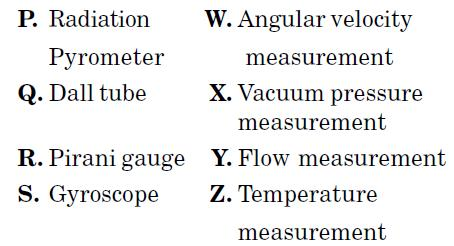Gyroscope Angular: Velocity

QUESTION: 4

A well of cross-sectional area aw is connected to an inclined tube of cross-sectional area at to form a differential pressure gauge as shown in the figure below. When p1 = p2 the common liquid level is denoted by A. When p1 > p2, the liquid level in the well is depressed to B, and the level in the tube rises by l along its length such that the difference in the tube and well levels is hd.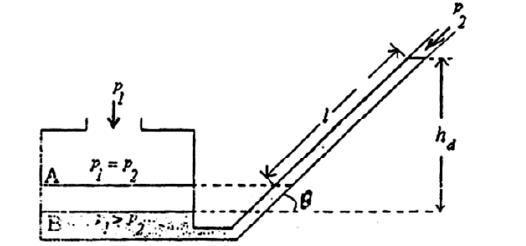The angle of inclination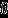of the tube with the horizontal is:

Solution: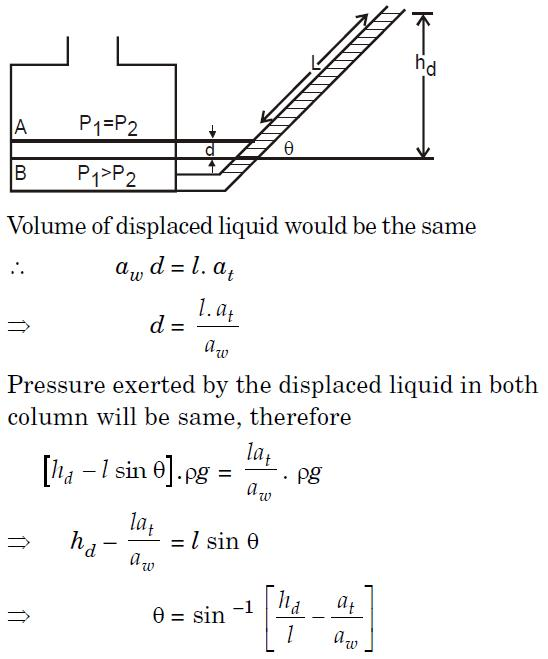QUESTION: 5

The diameter of an aluminium sphere is found to be 50 mm at atmospheric pressure of 105 Pa. The bulk modulus for aluminium is 68.6 GPa. The change in the diameter of the sphere in microns, when placed in a vacuum is

Solution:

We know Bulk Modulus =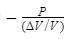⇒ 68.6 x 109 =⇒ 1 - (d' / d )3 = 1.45 x 10-3

⇒ d’ - d = 0.024 μ m

QUESTION: 6

A pressure sensor has the following specifications. Sensitivity at the design temperature

= 10 V/MPa, Zero drift = 0.01 V/°C, Sensitivity drift = 0.01 (V/MPa)/°C When the sensor is used in an ambient 20°C above the design temperature, the output from the device is 7.4 V. The true value of the pressure will be

Solution: Output voltage,

V0 = (0.01 V/°C) × 20 + [0.01 (V/MPa)]. 20 Ptrue + 10 Ptrue

or 7.4 = 0.2 + 0.01 × 20 P + 10 P

or P = 0.71 MPa.

QUESTION: 7

A piezoelectric type 50 MPa full-scale pressure sensor with built-in electronics has a sensitivity of 100 mV per MPa. If this sensor is subjected to a static pressure of 10 MPa then its output will be

Solution:

Output = Sensitivity × static pressure applied

= (100 mV/MPa) × (10 MPa)

= 1000 mV

QUESTION: 8

A piezoelectric type pressure sensor has a sensitivity of 1 mV/kPa and bandwidth of

300 Hz to 300 kHz. For a constant (dc) pressure of 100 kPa, the steady-state output of the sensor in millivolt is

Solution:

For constant (dc) pressure, the piezoelectric sensor will not respond, so the output voltage is 0 Volt.

QUESTION: 9

In which of the following categories is thin plate diaphragm included?

Solution: Primary transducers are devices that convert measurand into mechanical quantities. Diaphragm converts pressure into displacement which is a mechanical quantity.
QUESTION: 10

Which of the following devices convert pressure to displacement?

Solution: Both diaphragm and capsule convert pressure into displacement which can be measured using indicating instruments. Displacement will be proportional to applied pressure.
QUESTION: 11

What is the difference between water and transformer oil as a manometric liquid?

Solution: Both water and transformer oils are used in small pressure differential applications, but the difference is that water has problems related to relatively faster evaporation.
QUESTION: 12

Which of the following is detected using manometer devices?

Solution: In manometer devices, pressure difference between manometric liquid and measuring liquid is obtained.
QUESTION: 13

Which of the following conversions take place in bourdon tubes?Options

Solution:

In bourdon tubes converts input pressure into displacement and displacement of the needle will be directly proportional to input pressure.

QUESTION: 14